# python ppt.pptx

30 de May de 2023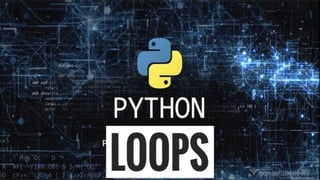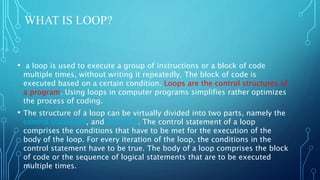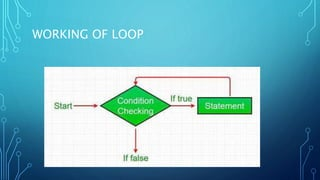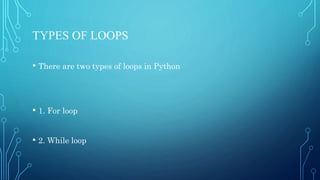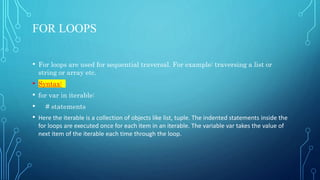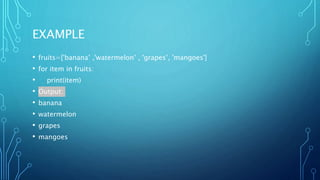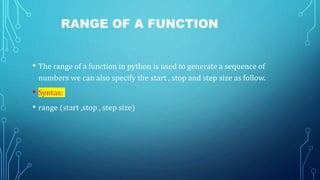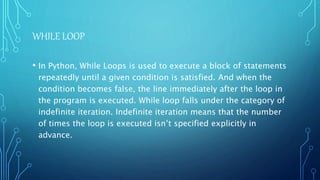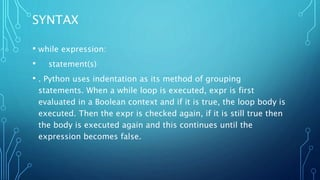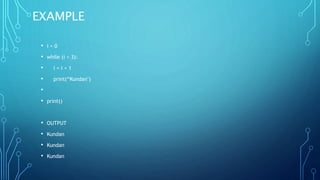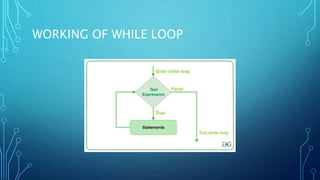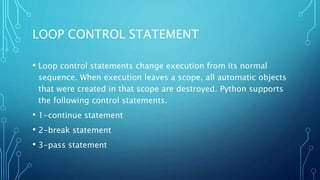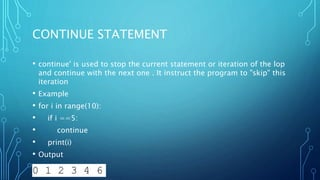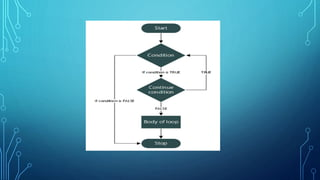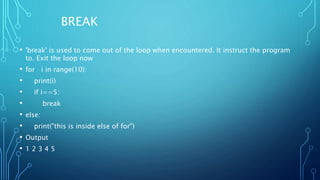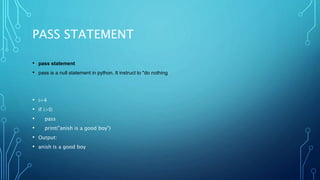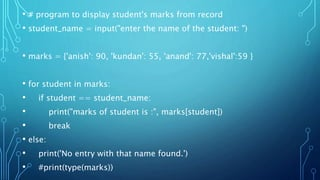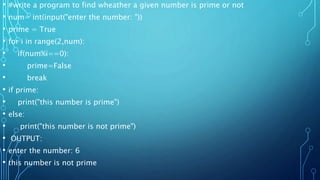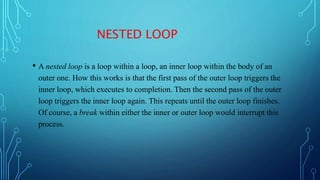1 de 24

### python ppt.pptx

• 2. WHAT IS LOOP? • a loop is used to execute a group of instructions or a block of code multiple times, without writing it repeatedly. The block of code is executed based on a certain condition. Loops are the control structures of a program. Using loops in computer programs simplifies rather optimizes the process of coding. • The structure of a loop can be virtually divided into two parts, namely the control statement, and the body. The control statement of a loop comprises the conditions that have to be met for the execution of the body of the loop. For every iteration of the loop, the conditions in the control statement have to be true. The body of a loop comprises the block of code or the sequence of logical statements that are to be executed multiple times.
• 4. TYPES OF LOOPS • There are two types of loops in Python • 1. For loop • 2. While loop
• 5. FOR LOOPS • For loops are used for sequential traversal. For example: traversing a list or string or array etc. • Syntax: • for var in iterable: • # statements • Here the iterable is a collection of objects like list, tuple. The indented statements inside the for loops are executed once for each item in an iterable. The variable var takes the value of next item of the iterable each time through the loop.
• 6. EXAMPLE • fruits=['banana’ ,'watermelon’ , 'grapes’, 'mangoes'] • for item in fruits: • print(item) • Output: • banana • watermelon • grapes • mangoes
• 7. RANGE OF A FUNCTION • The range of a function in python is used to generate a sequence of numbers we can also specify the start , stop and step size as follow. • Syntax: • range (start ,stop , step size)
• 8. • for i in range(10): • print(i) • else: • print("this is inside else of for") • Output: • 0 • 1 • 2 • 3 • 4 • 5 • 6 • 7 • 8 • 9
• 9. WHILE LOOP • In Python, While Loops is used to execute a block of statements repeatedly until a given condition is satisfied. And when the condition becomes false, the line immediately after the loop in the program is executed. While loop falls under the category of indefinite iteration. Indefinite iteration means that the number of times the loop is executed isn’t specified explicitly in advance.
• 10. SYNTAX • while expression: • statement(s) • . Python uses indentation as its method of grouping statements. When a while loop is executed, expr is first evaluated in a Boolean context and if it is true, the loop body is executed. Then the expr is checked again, if it is still true then the body is executed again and this continues until the expression becomes false.
• 11. EXAMPLE • i = 0 • while (i < 3): • i = i + 1 • print(“Kundan") • • print() • OUTPUT • Kundan • Kundan • Kundan
• 13. LOOP CONTROL STATEMENT • Loop control statements change execution from its normal sequence. When execution leaves a scope, all automatic objects that were created in that scope are destroyed. Python supports the following control statements. • 1-continue statement • 2-break statement • 3-pass statement
• 14. CONTINUE STATEMENT • continue' is used to stop the current statement or iteration of the lop and continue with the next one . It instruct the program to "skip" this iteration • Example • for i in range(10): • if i ==5: • continue • print(i) • Output 0 1 2 3 4 6
• 16. BREAK • 'break' is used to come out of the loop when encountered. It instruct the program to. Exit the loop now • for i in range(10): • print(i) • if i==5: • break • else: • print("this is inside else of for") • Output • 1 2 3 4 5
• 18. PASS STATEMENT • pass statement • pass is a null statement in python. It instruct to "do nothing • i=4 • if i>0: • pass • print("anish is a good boy") • Output: • anish is a good boy
• 19. • # program to display student's marks from record • student_name = input("enter the name of the student: ") • marks = {'anish': 90, 'kundan': 55, 'anand': 77,'vishal':59 } • for student in marks: • if student == student_name: • print("marks of student is :", marks[student]) • break • else: • print('No entry with that name found.') • #print(type(marks))
• 20. • #write a multiplication table of a given number using for loop • numb = int(input("enter the number")) • for i in range(1,11):t • # print(str(numb),"X“ + str(i),"=",str(i*numb)) • print(f"{numb}X{i}={numb*i}") • Output: • enter the number5 • 5X1=5 • 5X2=10 • 5X3=15 • 5X4=20 • 5X5=25 • 5X6=30 • 5X7=35 • 5X8=40 • 5X9=45 • 5X10=50
• 21. • #multiplication table using while loop • num = int(input("enter the number:")) • i=0 • while i<=10: • • print(f"{num}X{i}={num*i}") • i +=1 • output: • enter the number:5 • 5X0=0 • 5X1=5 • 5X2=10 • 5X3=15 • 5X4=20 • 5X5=25 • 5X6=30 • 5X7=35 • 5X8=40 • 5X9=45 • 5X10=50
• 22. • #write a program to find wheather a given number is prime or not • num= int(input("enter the number: ")) • prime = True • for i in range(2,num): • if(num%i==0): • prime=False • break • if prime: • print("this number is prime") • else: • print("this number is not prime") • OUTPUT: • enter the number: 6 • this number is not prime
• 23. NESTED LOOP • A nested loop is a loop within a loop, an inner loop within the body of an outer one. How this works is that the first pass of the outer loop triggers the inner loop, which executes to completion. Then the second pass of the outer loop triggers the inner loop again. This repeats until the outer loop finishes. Of course, a break within either the inner or outer loop would interrupt this process.